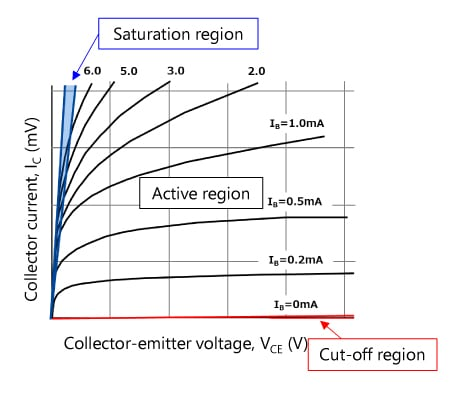# Basic idea of how to calculate the base current and input voltage of a bias resistor built-in transistor (BRT)

BRTs are generally used as switches. Ideally, their on-state voltage (collector-emitter voltage, VCE) should be as close to zero as possible.
The base current should be considered to achieve this condition.
To minimize VCE, BRTs are used in the saturation region shown in Figure 1. Typically, bipolar transistors are considered to provide an hFE of 100. However, since BRTs operate in the saturation region, it is necessary to assume that they provide an hFE of 10 to 20. This means that the base current should be 1/10th to 1/20th of the collector current.

Suppose that we need a VCE of 0.2 V and an IC of 10 mA. Here, the RN1402 (with R1=R2=10 kΩ) is used as a BRT. Look at the VCE(sat) – IC curves shown in the RN1402 datasheet (Figure 3). When hFE＝IC / IB＝20, VCE(sat) can be read as 0.05 V to 0.06 V at IC=10 mA. So, let’s assume these conditions.

The internal base current (Ib) of the basic BRT circuit shown in Figure 2 is:
Ib＝ IC / hFE = 10 mA / 20 = 0.5 mA
For the sake of simplicity, assume that the internal base voltage (Vbe) is equal to the commonly used value of 0.7 V. Then, IR2 flowing through R2 is calculated as follows:
IR2 = 0.7 V / 10 kΩ = 0.07 mA
Therefore, the center value of the BRT’s base current (IB) is:
IB = Ib + IR2 = 0.57 mA
The input voltage (VI) that provides an IB of 0.57 mA is calculated as follows:
VI = R1 * IB + Vbe = 10 kΩ * 0.57 mA + 0.7 = 6.4 V

It can therefore be considered that a VCE of 0.2 V and an IC of 10 mA can be obtained by applying an input voltage of 6.4 V or higher, although it is necessary to take device variations and temperature characteristics into consideration.

In practice, an input voltage (VI) higher than 6.4 V should be applied to the B terminal. The following paragraphs describe the operation of the BRT when VI > 6.4 V.

VCE(sat) specification in the datasheet (RN1401-RN1406)
Characteristic Symbol Test Circuit Test Condition Min Typ. Max Unit

Collector-emitter

saturation voltage

RN1401 to 1406 VCE(sat) IC = 5 mA, IB = 0.25mA
0.1 0.3 V

It is OK to apply a voltage higher than 6.4 V to the B terminal. Increasing the input voltage only brings the BRT into deeper saturation (i.e., decreases VCE(sat)). This causes the base-emitter voltage of the internal transistor to increase slightly, but not significantly. (For your reference, Figure 4 shows the VBE(sat)-IC curve (hFE=10) of the 2SC2712 that is equivalent to the internal transistor of the RN1402.) The base-emitter voltage varies only by several hundreds of millivolts in the collector current (IC) range in which BRTs are commonly used.
Since the base voltage hardly changes, an increase in the input voltage causes the voltage across R1 to increase, leading to an increase in the base current (ib). Saturation collector current, which is determined by load resistance and other external factors, hardly changes. Since only the base current increases, the hFE of the internal transistor decreases, causing the saturation level to increase (i.e., VCE(sat) to decrease).
Note that an increase in the saturation level might cause the time required to turn off the transistor to increase.
The maximum value is specified for the input voltage (VI). Also see the following FAQ entry.Figure 1 Example of operating regions of a bipolar transistor (2SC2712)